# 机器学习【八】数据预处理、降维、特征提取及聚类

#导入numpy
import numpy as np
#导入画图工具
import matplotlib.pyplot as plt
#导入数据集生成工具
from sklearn.datasets import make_blobs
X,y = make_blobs(n_samples=40,centers=2,random_state=50,cluster_std=2)
#用散点图绘制数据点
plt.scatter(X[:,0],X[:,1],c=y,cmap=plt.cm.cool)
plt.show()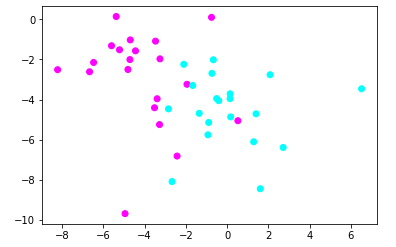【结果分析】

1.使用StandardScaler预处理数据

#导入StandardScaler
from sklearn.preprocessing import StandardScaler
#使用StandardScaler进行数据预处理
X_1 = StandardScaler().fit_transform(X)
#用散点图绘制预处理的数据点
plt.scatter(X_1[:,0],X_1[:,1],c=y,cmap=plt.cm.cool)
plt.show()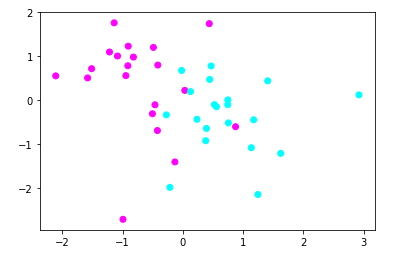【结果分析】

2.使用MinMaxScaler数据预处理

#导入MinMaxScaler
from sklearn.preprocessing import MinMaxScaler
#使用MinMaxScaler进行数据预处理
X_2 = MinMaxScaler().fit_transform(X)
#用散点图绘制预处理的数据点
plt.scatter(X_2[:,0],X_2[:,1],c=y,cmap=plt.cm.cool)
plt.show()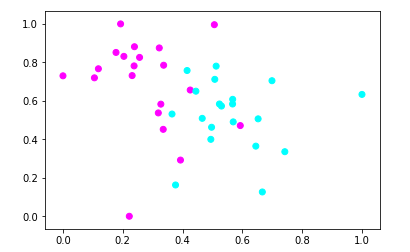【结果分析】

3.使用RobustScaler数据预处理

#导入RobustScaler
from sklearn.preprocessing import RobustScaler
#使用RobustScaler进行数据预处理
X_3 = RobustScaler().fit_transform(X)
#用散点图绘制预处理的数据点
plt.scatter(X_3[:,0],X_3[:,1],c=y,cmap=plt.cm.cool)
plt.show()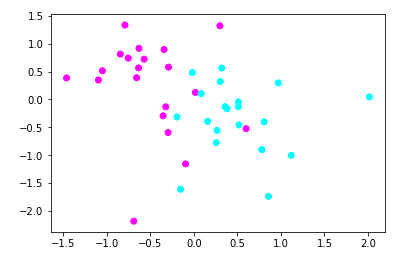【结果分析】

4.使用Normalizer数据预处理

#导入Normalizer
from sklearn.preprocessing import Normalizer
#使用Normalizer进行数据预处理
X_4 = Normalizer().fit_transform(X)
#用散点图绘制预处理的数据点
plt.scatter(X_4[:,0],X_4[:,1],c=y,cmap=plt.cm.cool)
plt.show()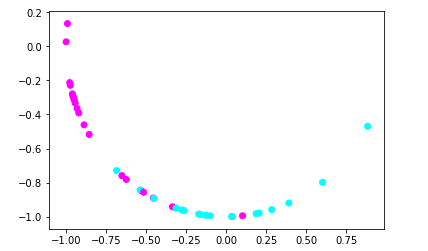#导入红酒数据集
#导入MLP神经网络
from sklearn.neural_network import MLPClassifier
#导入数据集拆分工具
from sklearn.model_selection import train_test_split
#建立训练集和测试集
X_train,X_test,y_train,y_test = train_test_split(wine.data,wine.target,random_state=62)
#打印数据形态
print(X_train.shape,X_test.shape)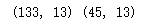【结果分析】

1.用训练集训练一个MLP神经网络，看看在测试集中的得分

#设定MLP神经网络的参数
mlp = MLPClassifier(hidden_layer_sizes=[100,100],max_iter=400,random_state=62)
#使用MLP拟合数据
mlp.fit(X_train,y_train)
#打印得分
print(mlp.score(X_test,y_test))
0.9333333333333333

2.预处理数据

#使用MinMaxScaler进行数据预处理
scaler = MinMaxScaler()
scaler.fit(X_train)
X_train_pp = scaler.transform(X_train)
X_test_pp = scaler.transform(X_test)
#重新训练模型
mlp.fit(X_train_pp,y_train)
#得分
print(mlp.score(X_test_pp,y_test))
1.0

【结果分析】

1.PCA主成分分析原理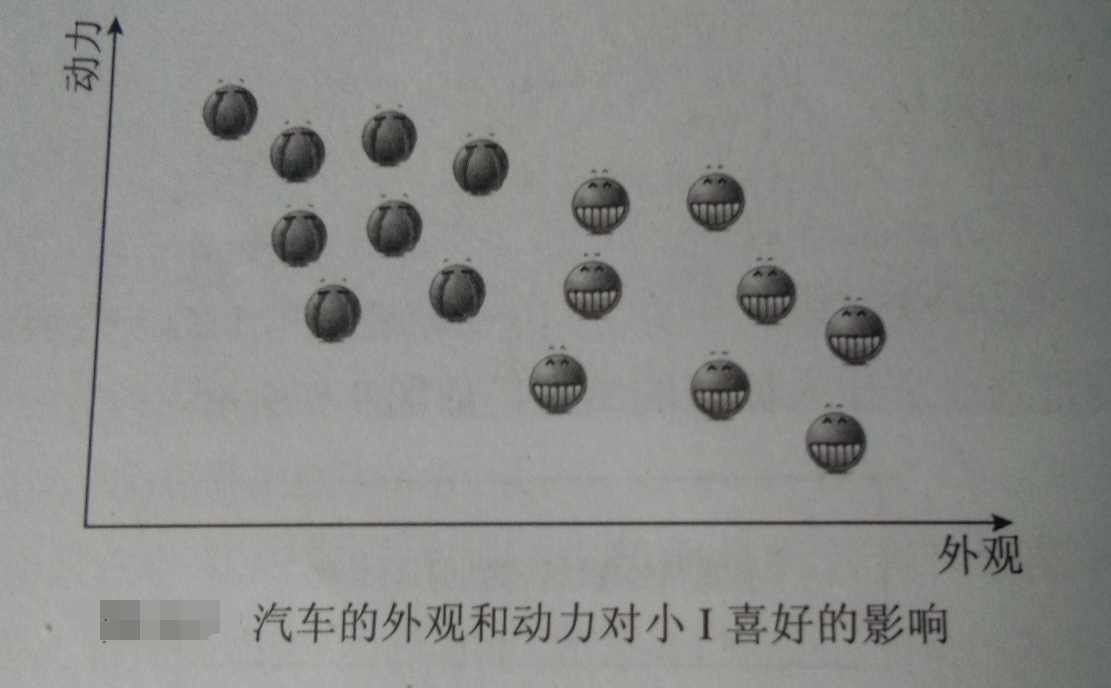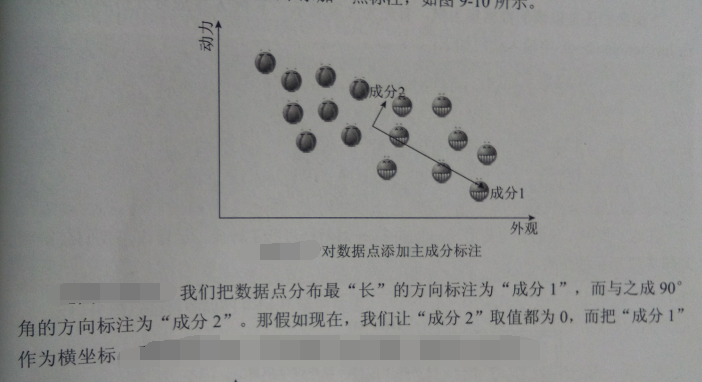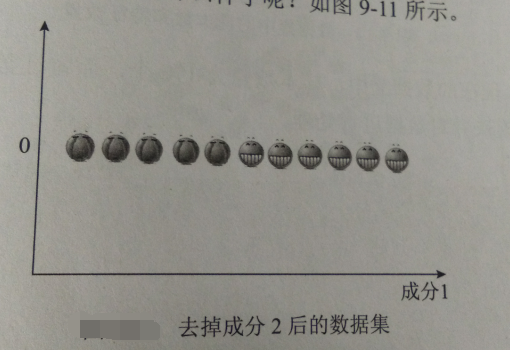• 超高维度数据
• 特征之间有非常强烈的相关性【比如，人口数据中，男性为1，女性为0，去掉其中任何一列，不会丢失任何信息，可以降维，以降低模型的复杂度】

#导入数据预处理工具
from sklearn.preprocessing import StandardScaler
#对红酒数据集预处理
scaler = StandardScaler()
X = wine.data
y = wine.target
X_scaled = scaler.fit_transform(X)
#打印处理后的数据集形态
print(X_scaled.shape)
(178, 13)

接下来导入PCA模块，并对数据进行处理

#导入PCA
from sklearn.decomposition import PCA
#设置生成分数量为2以便可视化
pca  = PCA(n_components=2)
pca.fit(X_scaled)
X_pca = pca.transform(X_scaled)
#打印主成分提取后的数据形态
print(X_pca.shape)
(178, 2)

#将三个分类中的主成分提取出来
X0 = X_pca[wine.target==0]
X1 = X_pca[wine.target==1]
X2 = X_pca[wine.target==2]
#绘制散点图
plt.scatter(X0[:,0],X0[:,1],c='b',s=60,edgecolor='k')
plt.scatter(X1[:,0],X1[:,1],c='g',s=60,edgecolor='k')
plt.scatter(X2[:,0],X2[:,1],c='r',s=60,edgecolor='k')
#设置图注
plt.legend(wine.target_names,loc='best')
plt.xlabel('component 1')
plt.ylabel('component 2')
plt.show()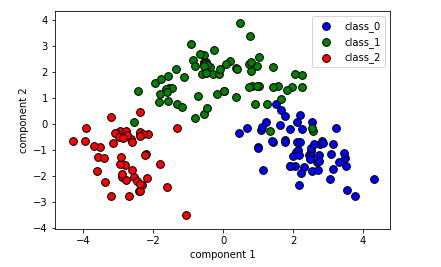【结果分析】

在之前的章节中，为了进行可视化，只能选取酒数据集的前两个特征，而去掉了其余的11个特征

#使用主成分绘制热度图
plt.matshow(pca.components_,cmap='plasma')
#纵轴为主成分数
plt.yticks([0,1],['component 1','component 2'])
plt.colorbar()
#横轴为原始特征数量
plt.xticks(range(len(wine.feature_names)),wine.feature_names,rotation=60,ha='left')
plt.show()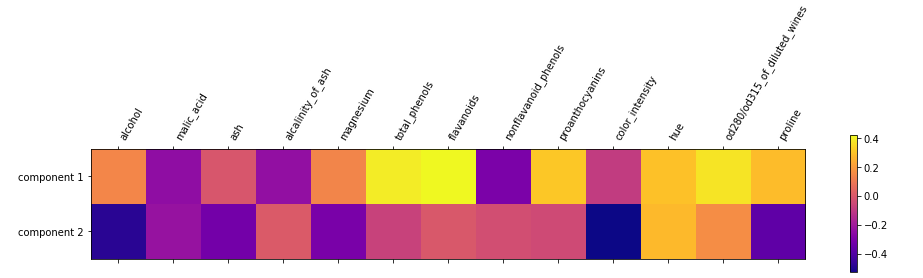【结果分析】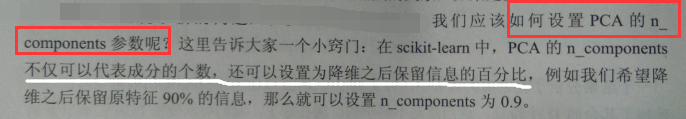1.PCA主成分分析法用于特征提取

#导入数据集获取工具from sklearn.datasets import fetch_lfw_people#载入人脸数据集faces = fetch_lfw_people(min_faces_per_person=20,resize=0.8)image_shape = faces.images.shape#将照片打印出来fig,axes = plt.subplots(3,4,figsize=(12,9),subplot_kw={'xticks':(),'yticks':()})for target,image,ax in zip(faces.target,faces.images,axes.ravel()):    ax.imshow(image,cmap=plt.cm.gray)    ax.set_title(faces.target_names[target])plt.show()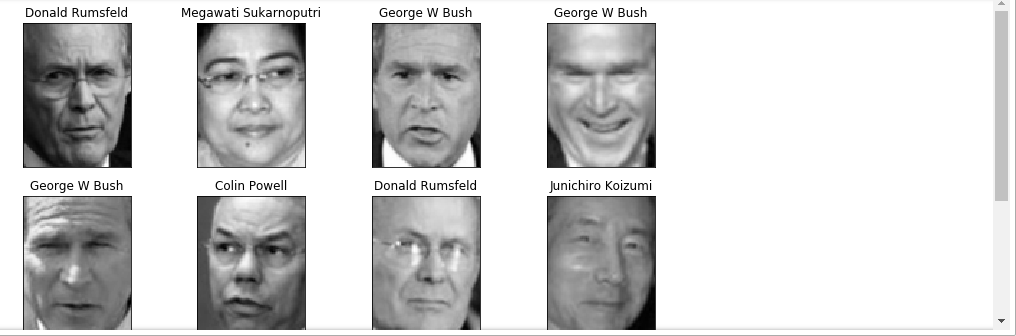#导入神经网络
from sklearn.neural_network import MLPClassifier
#对数据集拆分
X_train,X_test,y_train,y_test = train_test_split(faces.data/255,faces.target,random_state=62)
#训练神经网络
mlp=MLPClassifier(hidden_layer_sizes=[100,100],random_state=62,max_iter=400)
mlp.fit(X_train,y_train)
print(mlp.score(X_test,y_test))

0.7477064220183486

【结果分析】

在使用2个节点数为100的隐藏层时，神经网络的识别准确率只有0.74

2.使用一些方法来提升模型的表现

A.PCA中的数据白化功能

虽然每个人的面部特征由很大差异，但从像素级别观察图像，差异并不大

#使用白化功能处理人脸数据
pca = PCA(whiten=True,n_components=0.9,random_state=62).fit(X_train)
X_train_whiten = pca.transform(X_train)
X_test_whiten = pca.transform(X_test)
#打印白化后数据形态
print(X_train_whiten.shape)
(1306, 99)

【结果分析】

这里我们要求保留原始特征中90%的信息，所以n_components=0.9

可见，经过PCA白化处理后的数据成分为99个，远远小于原始特征数量

#使用白化后的数据训练神经网络
mlp.fit(X_train_whiten,y_train)
print(mlp.score(X_test_whiten,y_test))
0.7293577981651376

【结果分析】

B.非负矩阵分解用于特征提取

NMF， 也是一种无监督学习算法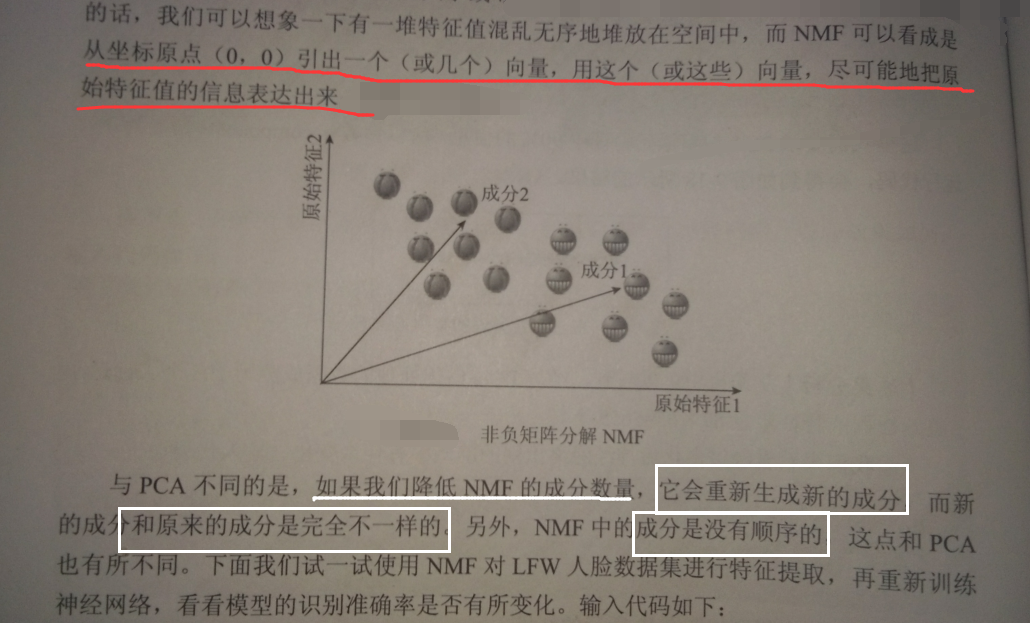#导入NMF
from sklearn.decomposition import NMF
nmf = NMF(n_components=105,random_state=62).fit(X_train)
X_train_nmf = nmf.transform(X_train)
X_test_nmf = nmf.transform(X_test)
#打印NMF处理后的数据形态
print(X_train_nmf.shape)
(1306, 105)

【结果分析】

NMF的n_components参数不支持使用浮点数，只能设置为正的整型数

#使用NMF处理后的数据训练神经网络
mlp.fit(X_train_nmf,y_train)
print(mlp.score(X_test_nmf,y_test))
0.7591743119266054

聚类算法

1.K均值算法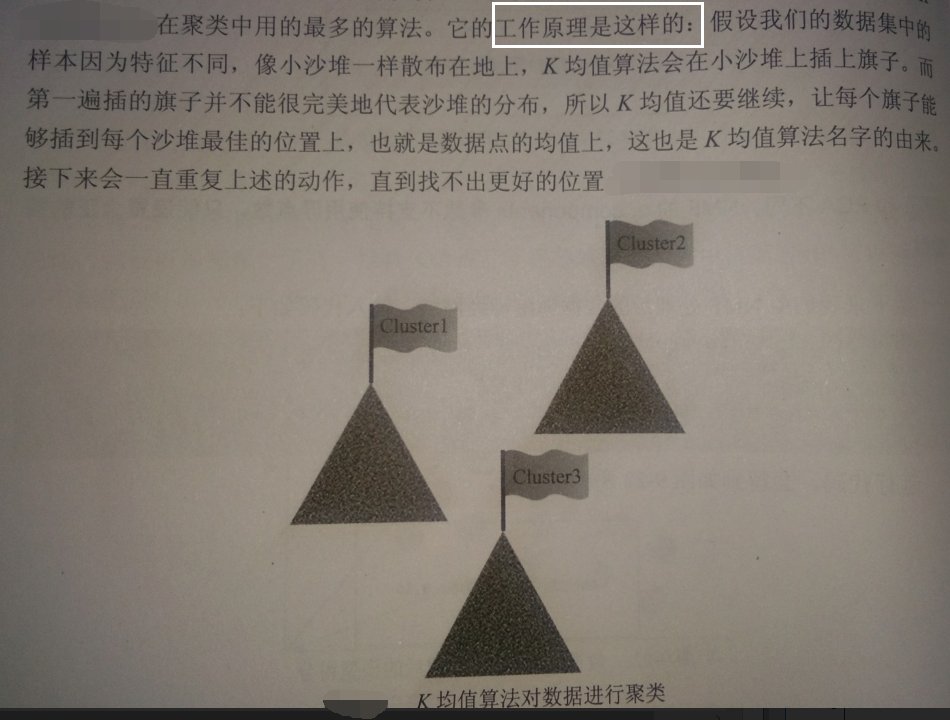#导入数据集生成工具
from sklearn.datasets import make_blobs
#生成分类数为1的数据集
blobs = make_blobs(random_state=1,centers=1)
X_blobs = blobs
#绘制散点图
plt.scatter(X_blobs[:,0],X_blobs[:,1],c='r',edgecolor='k')
plt.show()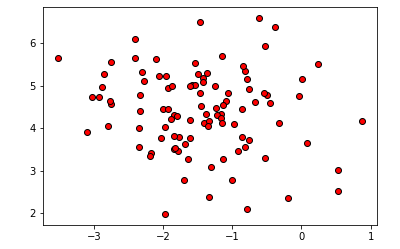【结果分析】

这段代码，主要是生成一坨没有类别的数据点

make_blobs的centers参数为1，因此这些数据都属于1类

#导入KMeans工具
from sklearn.cluster import KMeans
#要求KMeans将数据聚为3类
kmeans = KMeans(n_clusters=3)
#拟合数据
kmeans.fit(X_blobs)
#画图
x_min,x_max =  X_blobs[:,0].min() -0.5,X_blobs[:,0].max() +0.5
y_min,y_max =  X_blobs[:,1].min() -0.5,X_blobs[:,1].max() +0.5
xx,yy = np.meshgrid(np.arange(x_min,x_max,.02),np.arange(y_min,y_max,.02))
Z = kmeans.predict(np.c_[(xx.ravel(),yy.ravel())])
#将每个分类中的样本分配不同的颜色
Z = Z.reshape(xx.shape)
plt.figure(1)
plt.imshow(Z,interpolation='nearest',extent=(xx.min(),xx.max(),yy.min(),yy.max()),cmap=plt.cm.summer,aspect='auto',origin='lower')
plt.plot(X_blobs[:,0],X_blobs[:,1],'r.',markersize=5)
#用蓝色叉号表示聚类的中心
centroids = kmeans.cluster_centers_
plt.scatter(centroids[:,0],centroids[:,1],marker='x',s=150,linewidth=3,color='b',zorder=10)
plt.xlim(x_min,x_max)
plt.ylim(y_min,y_max)
plt.xticks(())
plt.yticks(())
plt.show()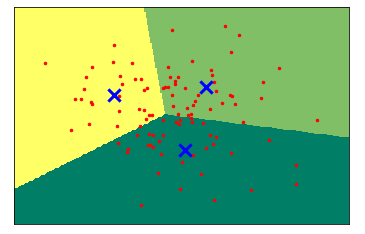【结果分析】

n_clusters=3，所以K均值将数据点聚为3类

#打印KMeans进行聚类的标签
print(kmeans.labels_)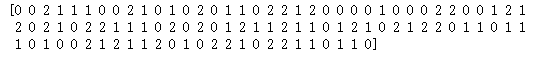【结果分析】

K均值对数据进行的聚类和分类有些类似，是用0、1、2三个数字来代表数据的类，并且存储在 .labels_ 属性中

2.凝聚聚类算法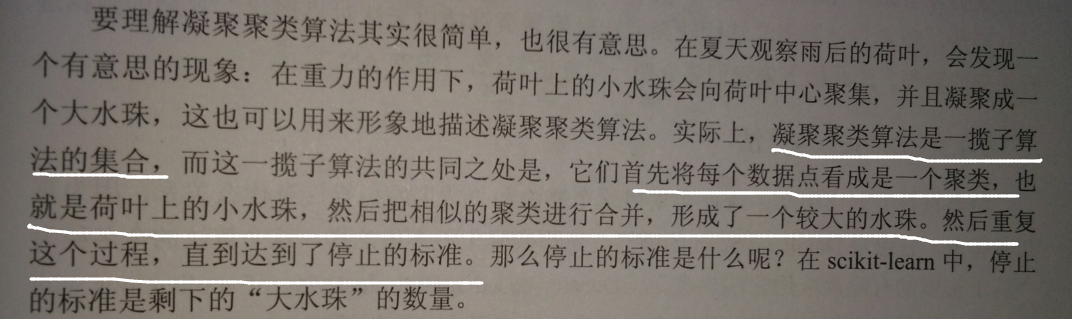#导入dendrogram和ward工具
from scipy.cluster.hierarchy import dendrogram,ward
#使用连线的方式可视化
ax = plt.gca()
plt.xlabel("sample index")
plt.ylabel("cluster distance")
plt.show()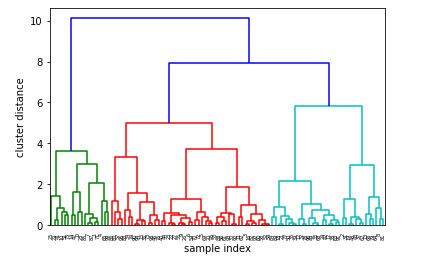【结果分析】

凝聚聚类算法是自下而上，不断合并相似的聚类中心，以便让类别变少

3.DBSCAN算法

基于密度的有噪声应用空间聚类

#导入DBSCAN
from sklearn.cluster import DBSCAN
db = DBSCAN()
#使用DBSCAN拟合数据
clusters = db.fit_predict(X_blobs)
#绘制散点图
plt.scatter(X_blobs[:,0],X_blobs[:,1],c=clusters,cmap=plt.cm.cool,s=60,edgecolor='k')
plt.xlabel("feature 0")
plt.ylabel("feature 1")
plt.show()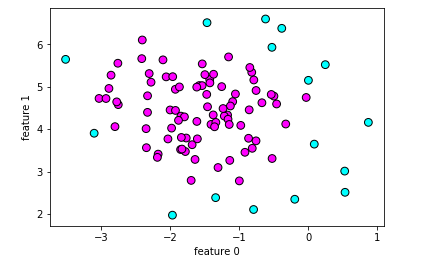#打印聚类个数
print(clusters)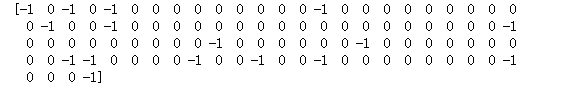【结果分析】

-1 代表该数据是噪声

eps参数，指考虑化入同一“坨”的样本距离多远

#设置DBSCAN参数eps=2
db_1 = DBSCAN(eps=2)
#使用DBSCAN拟合数据
clusters_1 = db_1.fit_predict(X_blobs)
#绘制散点图
plt.scatter(X_blobs[:,0],X_blobs[:,1],c=clusters_1,cmap=plt.cm.cool,s=60,edgecolor='k')
plt.xlabel("feature 0")
plt.ylabel("feature 1")
plt.show()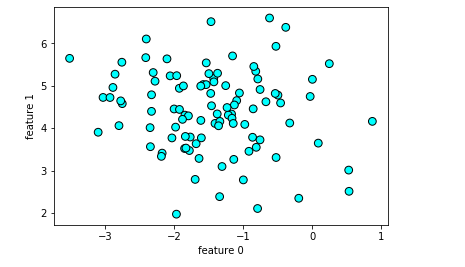min_samples参数，指定再某个数据点周围，被看成是聚类核心点的个数。越大，则核心数据点越少，噪声就越多

#设置DBSCAN的最小样本数为20
db_2 = DBSCAN(min_samples=20)
#使用DBSCAN拟合数据
clusters_2 = db_2.fit_predict(X_blobs)
#绘制散点图
plt.scatter(X_blobs[:,0],X_blobs[:,1],c=clusters_2,cmap=plt.cm.cool,s=60,edgecolor='k')
plt.xlabel("feature 0")
plt.ylabel("feature 1")
plt.show()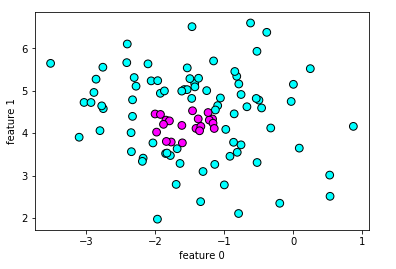【结果分析】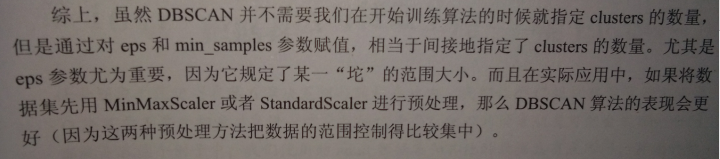posted @ 2019-05-06 19:48  远征i  阅读(6123)  评论(0编辑  收藏